Function Repository Resource:

# Intercepts

Compute the intercepts of a function with the coordinate axes

Contributed by: Wolfram|Alpha Math Team
 ResourceFunction["Intercepts"][expr,{x,y,…}] finds the intercepts of the expression expr with respect to the axis variables x,y,…. ResourceFunction["Intercepts"][expr,var,x] returns only the x-intercepts of the graph of expr.

## Details and Options

The first argument expr should be an equation (having head Equal).
ResourceFunction["Intercepts"] accepts the option "Constraints", expecting a logical combination of equalities and inequalities involving the axis variables.
Results of ResourceFunction["Intercepts"][expr,{x,y},] are returned in an association of the form <|x{{x1,0},{x2,0}},y|>.

## Examples

### Basic Examples (3)

Find the intercepts of a line:

 In:=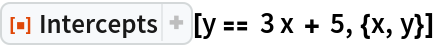Out=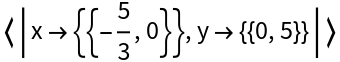In:=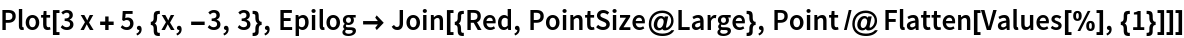Out=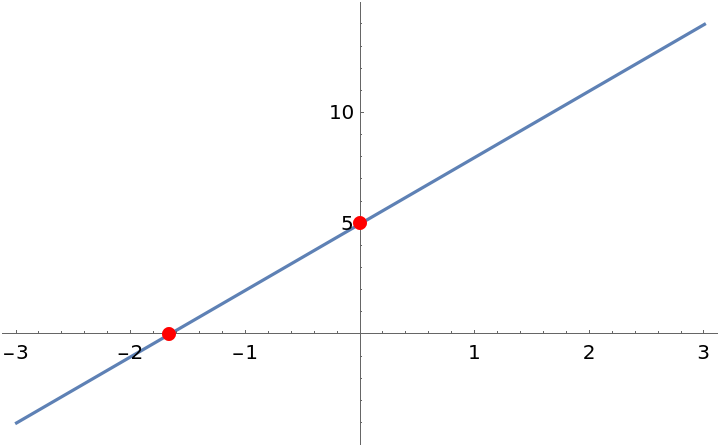Find the intercepts of a parabola:

 In:=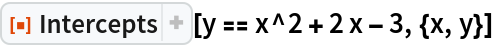Out=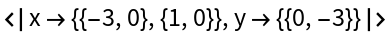In:=Out=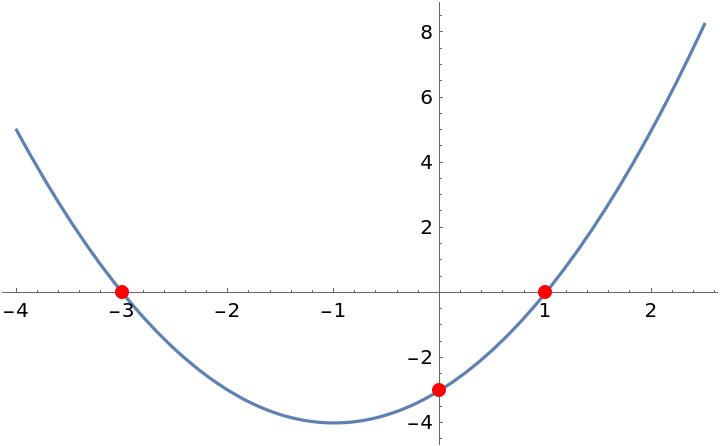Find the intercepts of an implicitly-defined circle:

 In:=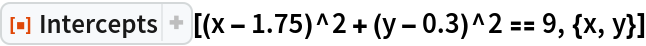Out=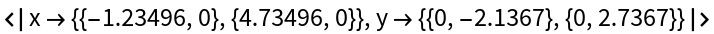In:=Out=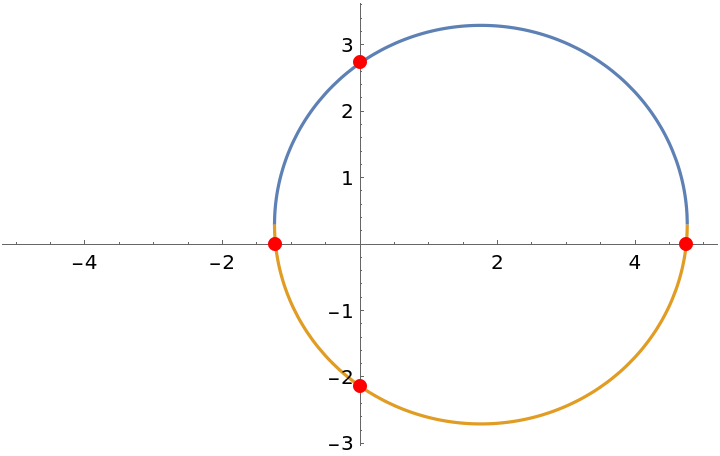### Scope (1)

Intercepts[expr,] can handle the use of symbolic parameters in expr:

 In:=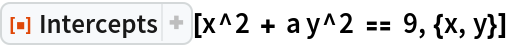Out=### Options (2)

Use the "Constraints" option to restrict the results returned:

 In:=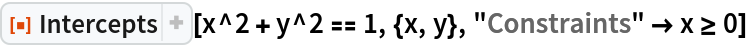Out=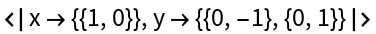With the default setting "Constraints" True, all results are included:

 In:=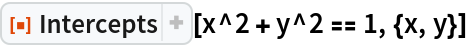Out=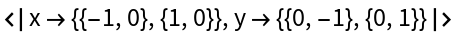## Publisher

Wolfram|Alpha Math Team

## Version History

• 10.0.0 – 23 March 2023
• 9.0.0 – 20 August 2020
• 8.0.0 – 24 January 2020
• 7.0.0 – 06 September 2019
• 6.0.0 – 12 June 2019
• 5.0.0 – 11 June 2019
• 4.0.0 – 11 June 2019
• 3.0.0 – 11 June 2019
• 2.0.0 – 11 June 2019
• 1.0.0 – 01 February 2019

## Author Notes

To view the full source code for Intercepts, run the following code:

FileNameJoin[ReplacePart[FileNameSplit[FindFile["ResourceFunctionHelpers`"]], -1 "Intercepts.wl"]] // SystemOpen